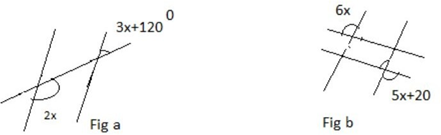# ICSE Class 8 Maths Important Questions

The ICSE Class 8 Maths important questions are prepared by the subject experts to help students in their exam preparation. The questions are created based on the latest syllabus of class 8 Maths. Students get familiar with the essential topics of each chapter of Maths. They can evaluate their performance and preparations in Class 8 Maths by practising ICSE  important questions. It helps them in enhancing their self-confidence as well. While framing the important questions, all the essential concepts and topics of Class 8 Maths are covered in order to help students improve their conceptual learning.

Solving these important questions in Maths will help students to make their concepts strong so that they can attempt any type of question in the exam. They will revise all the formulas and crucial concepts that need to be focused on more. It makes the exam preparation easier for the students.

Students should practise all the ICSE Class 8 important questions mentioned below to revise the entire ICSE Class 8 Maths Syllabus in a short duration of time.

1. Anil has 5Rs, 2Rs and 1Rs coins in the ratio 1:2:5 by numbers; if the total value of all the coins is Rs 35.2, find the number of each type of coin.
2. The perimeter of a triangle is 8-9y+6 = 0, and its two sides are 4-7y and 4+14, find its third side.
3. If a+b = 8and ab = 15, find a3=b3
4. Factories 10+6 – 5
5. Given the universal set = {-7,-3,-1,0,5,6,8,9} find
1. A = {x:x<2}
2. B = {y:y =2n+3, n belongs to N}
6. State the statements of inequalities.
7. Find the two consecutive even natural numbers such that the sum of their squares is 62.
8. For what value of x the lines will be parallel to each other9. Prove that the angles of a triangle are equal to two right angles.
10. The simple interest on a certain sum of money is the sum in 4 years. Find the Rate percentage charged.
11. Calculate the difference between the compound interest and simple interest on Rs 9500 in three years and 15% per annum.
12. Two pipes take x minutes and (x+3) minutes, respectively, to fill a cistern in 7 minutes; find the value of x.
13. Solve and graph the equation set on a number line: 5x+6 >8x-16;x €N
14. Construct an isosceles right-angled triangle whose hypotenuse is 7.5 cm. b) AB = BC = CA and the altitude AD = 5cm
15. Prove that the diagonals of a parallelogram bisect each other.
16. An exterior and an interior angle of a regular polygon are in the ration 3:6. Find the number of sides in the polygon.
17. An empty cistern can be filled by two pipes, A and B minutes and 20 minutes, respectively, and the full cistern can be emptied by a third pipe C, in 10 minutes. If all the pipes are turned on at the same time, in how much time will the empty cistern be full?
18. The price of a pen is increased by 35 %. By what per cent must the shopkeeper finally have the pen to a customer with an initial price of 250 Rs
19. Solve and plot the graph
1. 2x-3y = -6
2. 2x-y =7
20. In a parallelogram proves that opposite sides are equal, opposite angles are equal, and each diagonal bisects the parallelogram.
21. Construct a quadrilateral ABCD; IF AB = 6cm, BC = 6cm AD= 4CM and <BCD = 750

### Why should practise the ICSE Class 8 Maths Important Questions

Here, we have listed a few advantages of solving important Maths questions. After solving them, ICSE Class 8 students will understand how helpful these questions are for exam preparation.

• It boosts the confidence of the students, which in turn helps them score good marks.
• It helps them to analyze their preparation and improve on their weak topics.
• It covers all the important numerical problems that might be asked in the final exam.
• After completing the prescribed syllabus, students should solve these important questions for more practice.

Students can access the ICSE important questions for Class 8 for other subjects as well. Keep learning and stay tuned with BYJU’S for the latest update on ICSE/ISC/CBSE/State Board/Competitive Exams. Also, download the BYJU’S App for interactive study videos.